# Electronics and Communication Engineering - Automatic Control Systems

### Exercise :: Automatic Control Systems - Section 6

41.

Assertion (A): Integral control action is sometimes called reset control.

Reason (R): Reset rate is expressed as number of repeats per unit time.

 A. Both A and R are correct and R is correct explanation of A B. Both A and R are correct but R is not correct explanation of A C. A is correct but R is wrong D. R is correct but A is wrong

Explanation:

No answer description available for this question. Let us discuss.

42.

The polar plot in the given figure is for G(jω) =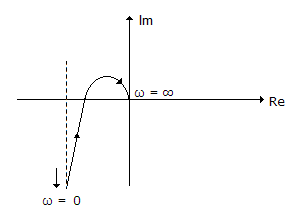A.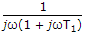B.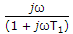C.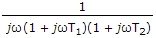D.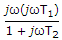Explanation:

No answer description available for this question. Let us discuss.

43.

In some on-off controllers a differential gap is provided

 A. to make the manufacture process easier B. to prevent too often frequent operation of controller C. both (a) and (b) D. neither (a) nor (b)

Explanation:

No answer description available for this question. Let us discuss.

44.

If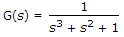there are

 A. two poles of G(s) in the left half plane B. two poles of G(s) in the right half plane C. three poles of G(s) in the right half plane D. four poles of G(s) in the right half plane

Explanation:

No answer description available for this question. Let us discuss.

45.

In Bode analysis the correction at corner frequency is 3 dB.

 A. True B. False